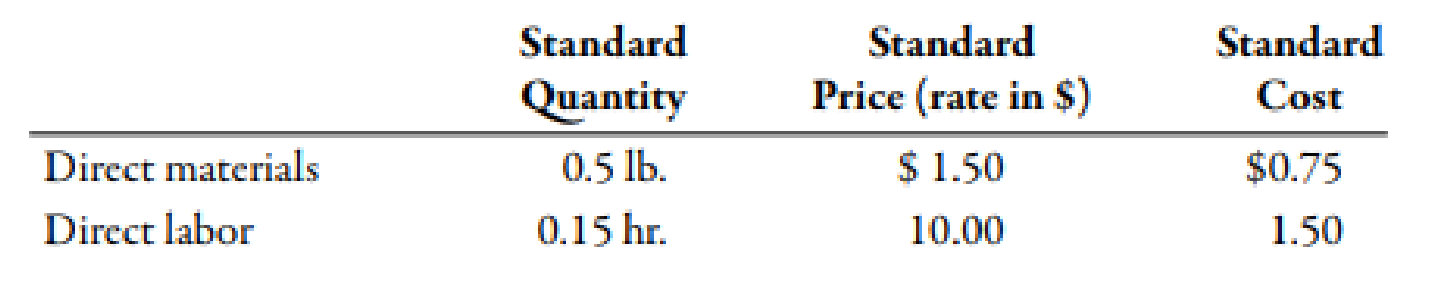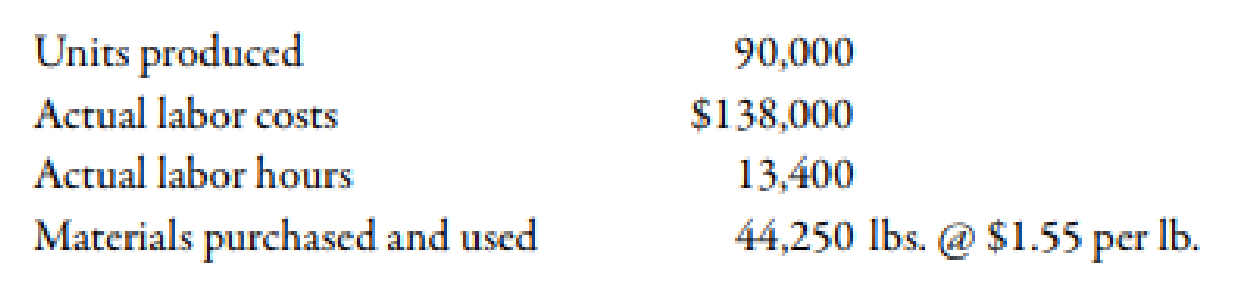Chapter 10, Problem 69P### Managerial Accounting: The Corners...

7th Edition
Maryanne M. Mowen + 2 others
ISBN: 9781337115773

#### Solutions

Chapter
Section### Managerial Accounting: The Corners...

7th Edition
Maryanne M. Mowen + 2 others
ISBN: 9781337115773
Textbook Problem
10 views

# Phono Company manufactures a plastic toy cell phone. The following standards have been established for the toys materials and labor inputs:During the first week of July, the company had the following results:The purchasing agent located a new source of slightly higher-quality plastic, and this material was used during the first week in July. Also, a new manufacturing layout was implemented on a trial basis. The new layout required a slightly higher level of skilled labor. The higher-quality material has no effect on labor utilization. Similarly, the new manufacturing approach has no effect on material usage. (Note: Round all variances to the nearest dollar.)Required: 1. CONCEPTUAL CONNECTION Compute the materials price and usage variances. Assuming that the materials variances are essentially attributable to the higher quality of materials, would you recommend that the purchasing agent continue to buy this quality, or should the usual quality be purchased? Assume that the quality of the end product is not affected significantly. 2. CONCEPTUAL CONNECTION Compute the labor rate and efficiency variances. Assuming that the labor variances are attributable to the new manufacturing layout, should it be continued or discontinued? Explain. 3. CONCEPTUAL CONNECTION Refer to Requirement 2. Suppose that the industrial engineer argued that the new layout should not be evaluated after only one week. His reasoning was that it would take at least a week for the workers to become efficient with the new approach. Suppose that the production is the same the second week and that the actual labor hours were 13,200 and the labor cost was $132,000. Should the new layout be adopted? Assume the variances are attributable to the new layout. If so, what would be the projected annual savings? 1. To determine Calculate the value of material price variance and material usage variance. Identify whether the plant manger could continue to purchase this quality product or purchase the usual quality. Explanation Variance: The amount obtained when actual cost is deducted from budgeted cost is known as variance. Variance is calculated to find whether the cost is over applied or under applied. Use the following formula to calculate material price variance: Material Price Variance=[(Actual Price×Actual Quantity)(Standard Price×Actual Quantity)] Substitute$1.55 for actual price, 44,250 units for actual quantity and $1.50 for standard price in the above formula. Material Price Variance=($1.55×44,250)($1.50×44,250)=$2,213(U)

Therefore, the material price variance is $2,213(U). Use the following formula to calculate material usage variance: Material Usage Variance=(Standard Price×Actual Quantity)(Standard Price×Standard Quantity1) Substitute$1

2.

To determine

Calculate the value of labor rate variance and labor efficiency variance. Identify whether the labor variances are attributable to the new manufacturing process should be continued or discontinued.

3.

To determine

Calculate the value of labor rate variance and labor efficiency variance. Identify whether the new process should be adopted.

### Still sussing out bartleby?

Check out a sample textbook solution.

See a sample solution

#### The Solution to Your Study Problems

Bartleby provides explanations to thousands of textbook problems written by our experts, many with advanced degrees!

Get Started

#### Find more solutions based on key concepts